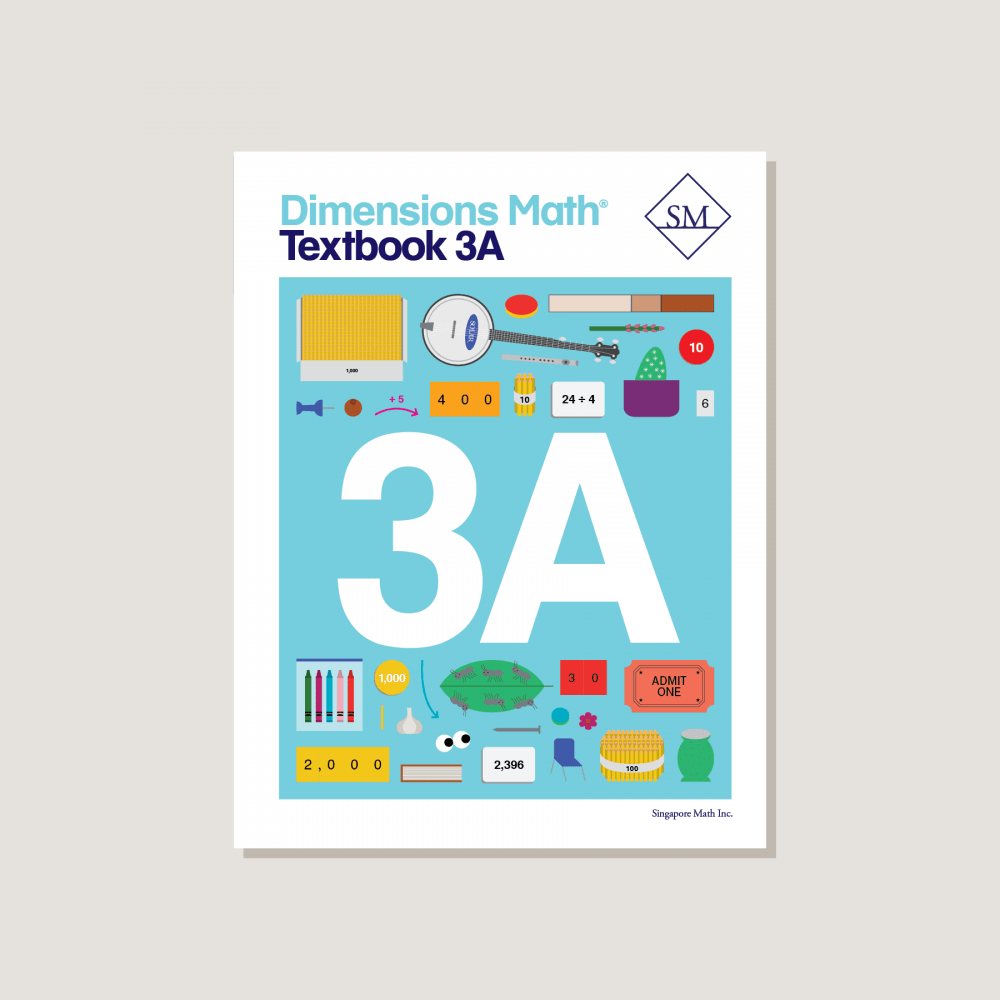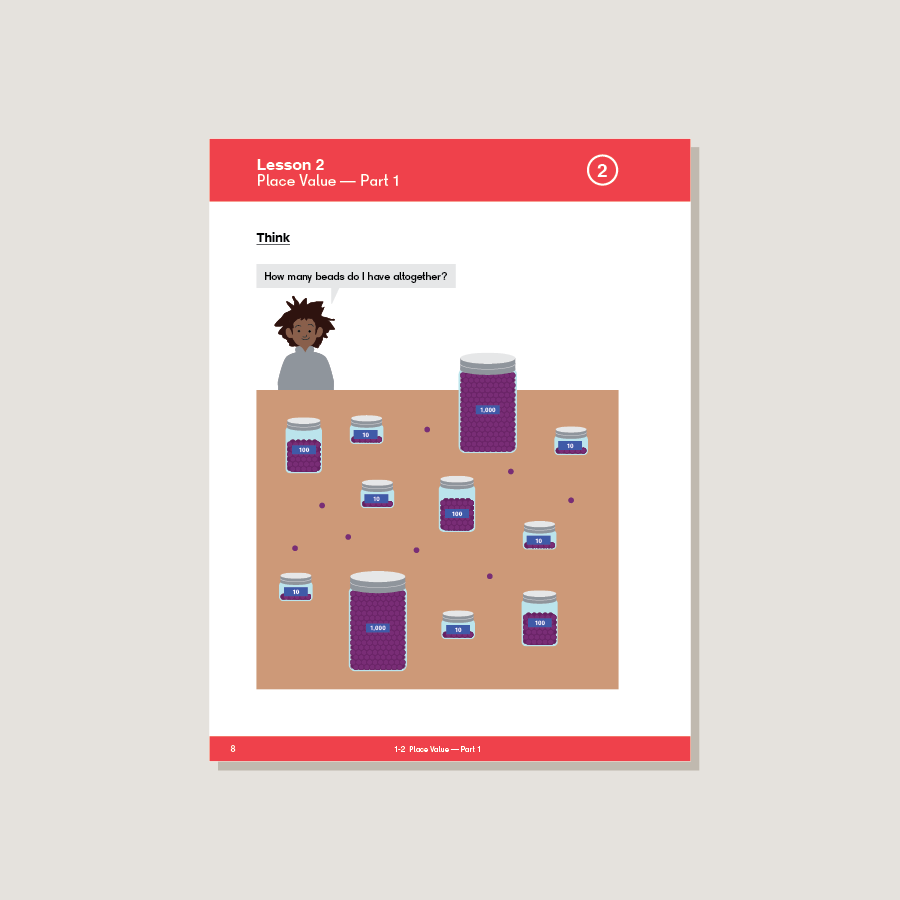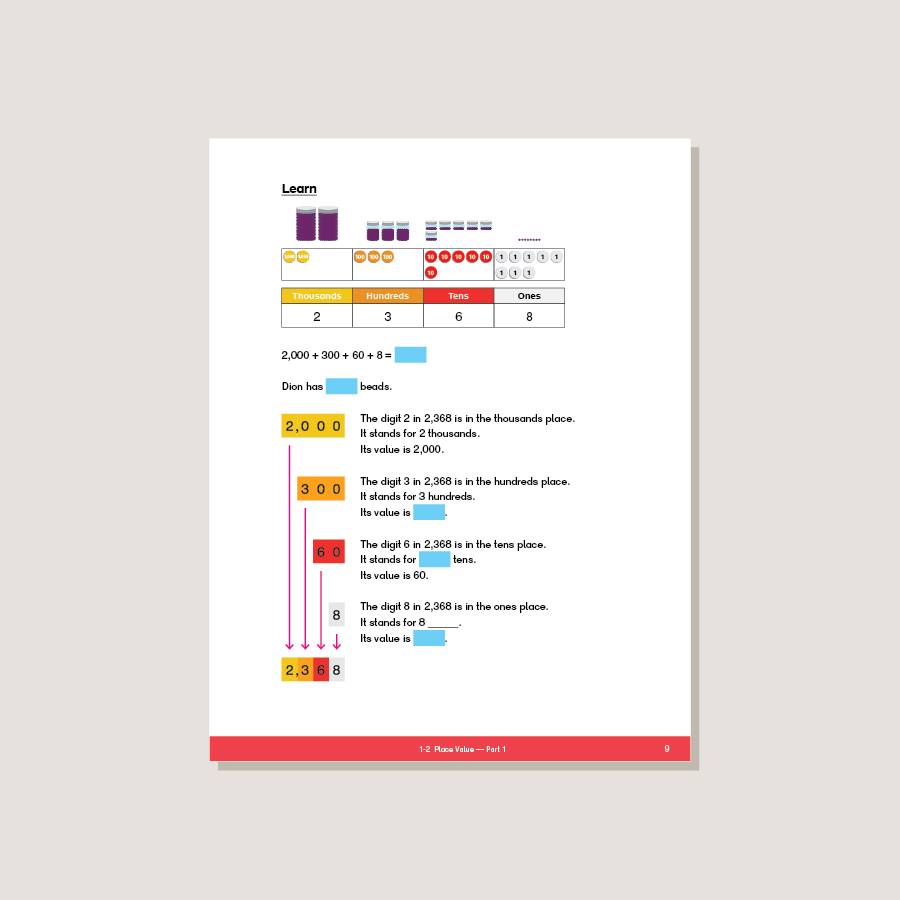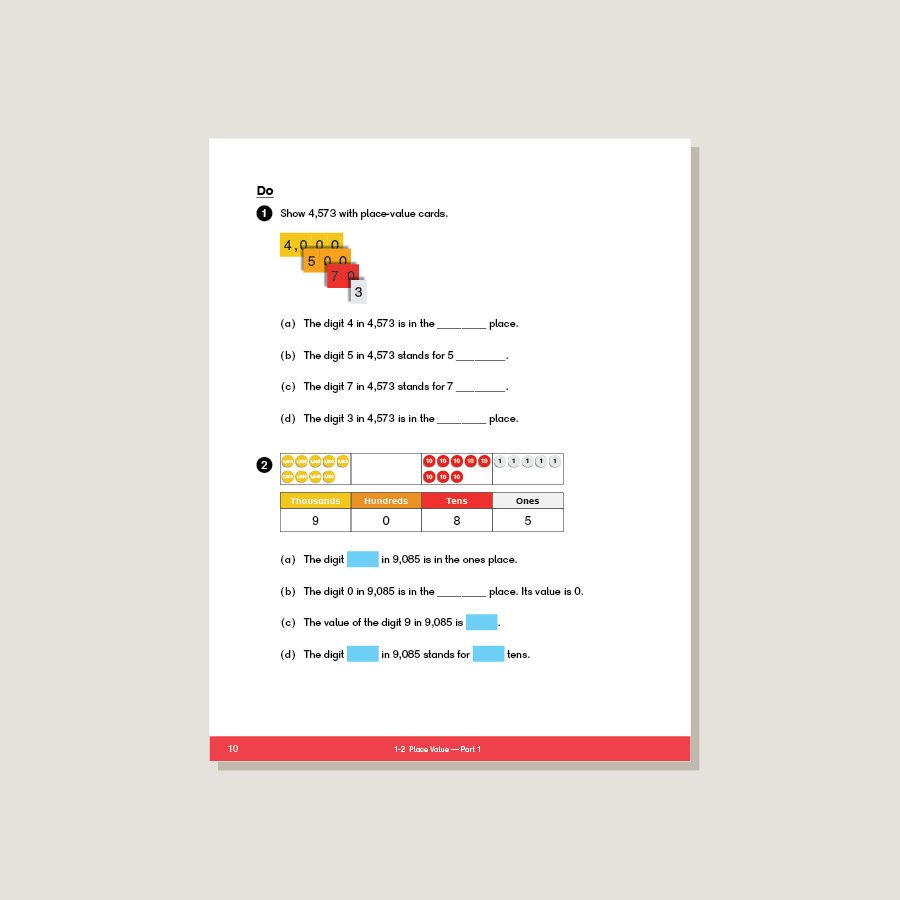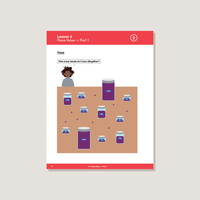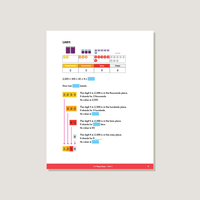# Dimensions Math Textbook 3A

\$13.70

Textbooks introduce concepts through logical sequencing. Lessons encourage discussion and help students reach fluency through engaging activities and strategies. Corresponding exercises in workbooks help students consolidate and practice new knowledge. Features & Components:
• Chapter Opener: Each chapter begins with an engaging scenario that stimulates student curiosity in new concepts. This scenario also provides teachers an opportunity to review skills.
• Think: With guidance from teachers, students solve a problem using a variety of methods.
• Learn: One or more solutions to the problem in Think are presented, along with definitions and other information to consolidate the concepts introduced in Think.
• Do: A variety of practice problems allow teachers to lead discussion or encourage independent mastery. These activities solidify and deepen student understanding of the concepts.
• Exercise: A pencil icon at the end of the lesson links to additional practice problems in the corresponding workbook.
• Practice: Periodic practice provides teachers with opportunities for consolidation, remediation, and assessment.
• Review: Two cumulative reviews provide ongoing practice of concepts and skills.
Note: Two textbooks (A and B) for each grade correspond to the two halves of the school year. Answer key not included. Soft cover.
SKU: DMT3A

ISBN: 9781947226081

Pagecount: 220

Dimensions: 8.5 x 11 x 0.75 in

Binding: Standard

Color: Color

Cover: Soft

Perforated: No

Sample Pages

Chapter 1: Numbers to 10,000
Lesson 1: Numbers to 10,000
Lesson 2: Place Value — Part 1
Lesson 3: Place Value — Part 2
Lesson 4: Comparing Numbers
Lesson 5: The Number Line
Lesson 6: Practice A
Lesson 7: Number Patterns
Lesson 8: Rounding to the Nearest Thousand
Lesson 9: Rounding to the Nearest Hundred
Lesson 10: Rounding to the Nearest Ten
Lesson 11: Practice B

Chapter 2: Addition and Subtraction — Part 1
Lesson 1: Mental Addition — Part 1
Lesson 2: Mental Addition — Part 2
Lesson 3: Mental Subtraction — Part 1
Lesson 4: Mental Subtraction — Part 2
Lesson 5: Making 100 and Making 1,000
Lesson 6: Strategies for Numbers Close to Hundreds
Lesson 7: Practice A
Lesson 8: Sum and Difference
Lesson 9: Word Problems — Part 1
Lesson 10: Word Problems — Part 2
Lesson 11: 2-Step Word Problems
Lesson 12: Practice B

Chapter 3: Addition and Subtraction — Part 2
Lesson 2: Subtraction with Regrouping — Part 1
Lesson 3: Subtraction with Regrouping — Part 2
Lesson 4: Estimating Sums and Differences — Part 1
Lesson 5: Estimating Sums and Differences — Part 2
Lesson 6: Word Problems
Lesson 7: Practice

Chapter 4: Multiplication and Division
Lesson 1: Looking Back at Multiplication
Lesson 2: Strategies for Finding the Product
Lesson 3: Looking Back at Division
Lesson 4: Multiplying and Dividing with 0 and 1
Lesson 5: Division with Remainders
Lesson 6: Odd and Even Numbers
Lesson 7: Word Problems — Part 1
Lesson 8: Word Problems — Part 2
Lesson 9: 2-Step Word Problems
Lesson 10: Practice
Review 1

Chapter 5: Multiplication
Lesson 1: Multiplying Ones, Tens, and Hundreds
Lesson 2: Multiplication Without Regrouping
Lesson 3: Multiplication with Regrouping Tens
Lesson 4: Multiplication with Regrouping Ones
Lesson 5: Multiplication with Regrouping Ones and Tens
Lesson 6: Practice A
Lesson 7: Multiply a 3-digit Number with Regrouping Once
Lesson 8: Multiplication with Regrouping More Than Once
Lesson 9: Practice B

Chapter 6: Division
Lesson 1: Dividing Tens and Hundreds
Lesson 2: Dividing a 2-digit Number by 2 — Part 1
Lesson 3: Dividing a 2-digit Number by 2 — Part 2
Lesson 4: Dividing a 2-digit Number by 3, 4, and 5
Lesson 5: Practice A
Lesson 6: Dividing a 3-digit Number by 2
Lesson 7: Dividing a 3-digit Number by 3, 4, and 5
Lesson 8: Dividing a 3-digit Number, Quotient is 2 Digits
Lesson 9: Practice B

Chapter 7: Graphs and Tables
Lesson 1: Picture Graphs and Bar Graphs
Lesson 2: Bar Graphs and Tables
Lesson 3: Practice
Review 2

A & B Books: Our programs divide the school year into two semesters. “A” level books are for the first half of the school year. “B” level books are for the second half of the school year. You need both “A” and “B” material for a complete school year.

Required Components: Textbooks, Workbooks, and Guides (either Home Instructor’s Guides or Teacher’s Guides) are all necessary components. These three elements each serve a unique function and work together to build math mastery.

Printouts: Dimensions Math PK-5 Resources

Recommended Manipulatives: Dimensions Math Grades PK-5 Recommended Manipulatives

Answer Key: Located in corresponding Teacher’s Guide

Dimensions Math At Home™ Videos

Invite a professional Singapore math teacher into your home classroom. This subscription of pre-recorded lessons covers all Textbook and Workbook material for an entire school year and can be done at your own pace.

Manipulatives

Manipulatives help students visualize and represent math concepts. Stock up on suggested items to deepen engagement during math activities and lessons.

Supplementary Math

Supplementary math helps students of all abilities: struggling, advanced, or on level. Find just the right addition to round out your core program.